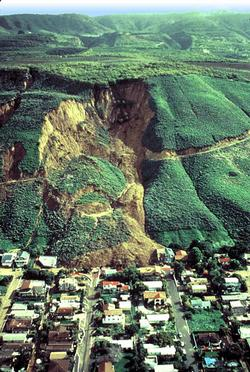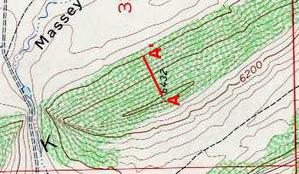# Conveying the relationship of hillslope to mathematical slopeAn instructor's guide to Calculating slope/gradient

by Dr. Jennifer M. Wenner, University of WI Oshkosh Geology, and Dr. Eric M. Baer, Highline Community College Geology.Many geoscience instructors find that, although students can tell them the concept of slope ("rise over run"), introductory geoscience students often misunderstand or have difficulty transferring the concept to real-world applications. Slope in the geosciences is often used in exercises that are associated with such context as topographic maps, groundwater, structural geology, etc. Transferring "rise over run" to tangible objects such as a hillslope or the water table can present problems for students. The information on this page is designed to help students with that transfer.

This page is designed to help students to understand the relationship of slope in the geosciences to calculation of mathematical slope. Before attempting this page, students should know how to read a topgraphic map and the contour lines on it. By the time the student finishes the page, s/he should:
1. Understand why s/he should know how to calculate slope in the geosciences
2. Become more comfortable reading and examining topographic maps and contour lines
3. Be able to calculate slope (in feet/foot or m/m or some sort of unitless number)
4. Be able to calculate % slope
Students should end up with a little more confidence in their abilities to apply the concept of slope to real-world problems.

## Why is it hard for students?

Students often struggle with the transfer of mathematical concepts to the context in which they are used, particularly if the concept is something tangible.

Ask a student how to calculate slope and most will simply reply "rise over run". But what does that mean when talking about the slope of a hillside or the water table? Often students need explicit help when it comes to transferring the ability to calculate slope on a graph to calculating slope of a surface. There are many places that students get stuck visualizing slope:1. understanding that "rise" represents change in elevation, and "run" represents distance.
2. understanding the concept of slope.
3. translating 2-D to 3-D and vice versa. Often in introductory geoscience we are examining something that is a three dimensional surface but just want them to calculate a slope along a given line. This makes the mathematics much more simple but may confuse the students.